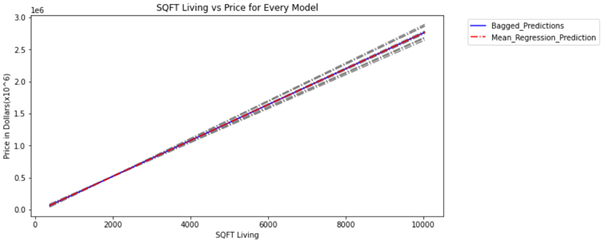# Bagging on Low Variance ModelsBagging on Low Variance Models. A curious case of bagging on simple linear regression

Bagging (also known as bootstrap aggregation) is a technique in which we take multiple samples repeatedly with replacement according to uniform probability distribution and fit a model on it. It combines multiple predictions to give a better prediction by majority vote or taking the aggregate of the predictions. This technique is effective on models which tend to overfit on the dataset (high variance models). Bagging reduces the variance without making the predictions biased. This technique acts as a base to many ensemble techniques so understanding the intuition behind it is crucial.

If this technique is so good, why do we use it only on models which show high variance? What happens when we use it with models which have low variance? Let us try to understand the underlying issue with the help of a demonstration.

We will be using bagging on decision tree to prove that bagging improves the accuracy for high variance models and compare it to bagging on simple linear regression which is biased depending on the dataset. Simple linear regression is biased when the predictor is not perfectly correlated to the target variable.

## Bias and Variance

We will be talking about Bias and Variance throughout the article so let us get an idea of what it is first.

### Bias

High bias refers to the oversimplification of the model. i.e. When we are unable to capture the true relation of the data. Our objective of creating a model is to capture the true nature of the data and predict based on the trend, which makes high bias an undesired phenomenon.

## Linear Regression VS Logistic Regression (MACHINE LEARNING)

Linear Regression VS Logistic Regression (MACHINE LEARNING). Linear Regression and Logistic Regression are two algorithms of machine learning and these are mostly used in the data science field.

Here, we will go over what Bias error and Variance error are, sources of these errors and how you can work to reduce these errors in your model.

## Regression: Linear Regression

Machine learning algorithms are not your regular algorithms that we may be used to because they are often described by a combination of some complex statistics and mathematics.

## What is Supervised Machine Learning

What is neuron analysis of a machine? Learn machine learning by designing Robotics algorithm. Click here for best machine learning course models with AI

## Pros and Cons of Machine Learning Language

AI, Machine learning, as its title defines, is involved as a process to make the machine operate a task automatically to know more join CETPA